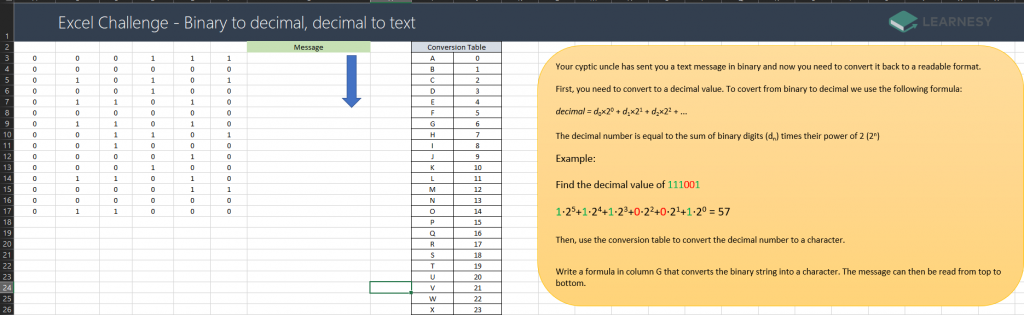Tackle 2024 with new knowledge – take advantage of Learnesys’ fall campaign on the All Courses package. Read more here.

# Excel challenge – Binary to text

Your cryptic uncle has sent you a text message in binary and now you need to convert it back to a readable format.

First, you need to convert to a decimal value. To convert from binary to decimal we use the following formula:

decimal = d0×20 + d1×21 + d2×22 + …

The decimal number is equal to the sum of binary digits (dn) times their power of 2 (2n)

Example:

Find the decimal value of 111001

1⋅25+1⋅24+1⋅23+0⋅22+0⋅21+1⋅20 = 57

Then, use the conversion table to convert the decimal number to a character.

Write a formula in column G that converts the binary string into a character. The message can then be read from top to bottom.Our solution:

=XLOOKUP(A3*2^5+B3*2^4+C3*2^3+D3*2^2+E3*2^1+F3*2^0;\$J\$3:\$J\$29;\$I\$3:\$I\$29)

#### 4 thoughts on “Excel challenge – Binary to text”

1.Fredrik Östman says:

=XLOOKUP(SUMPRODUCT(A3:F3;2^(6-COLUMN(A3:F3)));J:J;I:I)

2.Jeffrey Johns says:

=XLOOKUP(BIN2DEC(CONCAT(A3:F3));J:J;I:I)

3.Fredrik Östman says:

4.Jeffrey Johns says:

Thanks! And your 2^(6-COLUMN()) is pretty nifty.

### More blog postsTired of Googling?